## Problem statement

The sum of the squares of the first ten natural numbers is,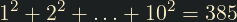The square of the sum of the first ten natural numbers is,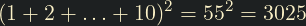Hence the difference between the sum of the squares of the first ten natural numbers and the square of the sum is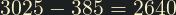.

Find the difference between the sum of the squares of the first one hundred natural numbers and the square of the sum.

## Thoughts

We can build on the solution to problem 1 to solve this. In problem 1 we summed natural numbers using this formula: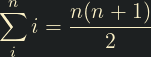We can derive from this a formula to sum the squares of natural numbers. I must confess I didn't come up with the derived formula myself: I searched for it online and found it on Brilliant.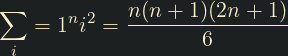## Solution

/* Implement the above formula */

unsigned long GetSumOfSquares(int limit)
{
return (limit * (limit + 1) * ((2 * limit) + 1)) / 6;
}

/* Use the formula to sum the natural numbers, and square the result */

#include <math.h>
unsigned long GetSquareOfSum(int limit)
{
return pow((limit * (limit + 1)) / 2, 2);
}

/* Subtract the difference */

#include <stdio.h>
int main()
{
int limit = 100;
printf("%lu", GetSquareOfSum(limit) - GetSumOfSquares(limit));
}